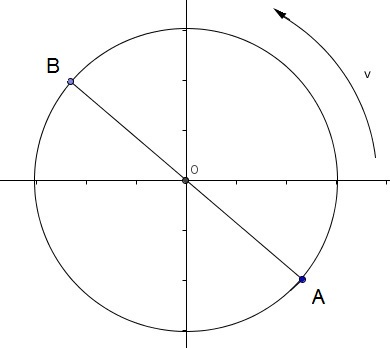# Free SAT II Physics Practice Questions with Solutions Uniform Circular Motion

 Free Sat Physics subject questions on uniform circular motion with detailed solutions and explanations Fig. 1 below is related to questions 1, 2 and 3.Fig1. - Uniform Circular Motion. Fig.1 above refer to a point moving along a circular path. What is the direction of the velocity of the moving point at A?Fig.1 above refer to a point moving along a circular path. What is the direction of the centripetal acceleration at point B?Fig.1 above refer to a point moving along a circular path. If BD is the diameter of the circle of rotation, then the velocities at A and B are A) equal in magnitude and have same direction B) equal in magnitude but have opposite direction C) not equal in magnitude but have the same direction D) not equal in magnitude but have opposite direction E) None of the above In a uniform circular motion, which of the following is constant? A) centripetal acceleration B) position C) velocity D) centripetal force E) speed F is the centripetal force of a sphere rotating along a circular path at speed s. The mass of the sphere is m and is at distance R from the center. What is the centripetal force of the same sphere if the distance R is doubled? A) 2 F B) F / 2 C) F / 4 D) 4 F E) F / 3 a is the centripetal acceleration of a sphere rotating along a circular path at speed s. The sphere is at distance R from the center. What is the centripetal acceleration of the same sphere if both distance R and speed s are doubled? A) a B) a / 4 C) a / 2 D) 2 a E) 4 a What is the ratio s1 / s2 where s1 and s2 are the speeds of two satellites placed in stable circular orbits of radius R and 2R respectively? A) 2 B) √2 C) 1 / 2 D) 1 / √2 E) 2 √2 What is the centripetal force acting on a car of mass 2000 Kg turning around a circular bend of radius 50 meters at the constant speed of 36 km/hr? A) 4,000 N B) 400 N C) 72,000 N D) 10,000 N E) 40 N What is the period of a point moving along a circular path of radius 5 cm at the linear speed of 200π cm / minutes? A) 10 s B) 300 s C) 180 s D) 40 se E) 3 s A car is being driven on a road having two distant circular bends B1 and B2 of radius R and 3R respectively. If s1 is the speed of the car at the bend B1 and s2 is the speed at the bend B2, what should the ratio s1/s2 be so that the centripetal forces at both bends are equal? A) 1 B) √3 C) 1 / √3 D) 1 / √2 E) √2 Answers to the Above questions D E B E B D B A E C